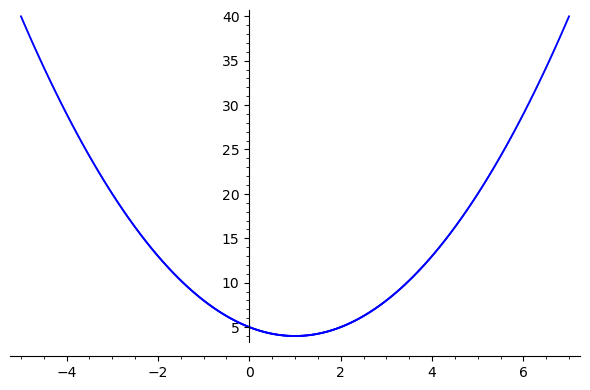# Argand diagram plot

HI

T=[-sqrt(1/2*sqrt(13) + 1) - I*sqrt(1/2*sqrt(13) - 1),
-sqrt(1/2*sqrt(13) + 1) + I*sqrt(1/2*sqrt(13) - 1),
-sqrt(1/2*sqrt(13) + 1) +I*sqrt(1 + sqrt(13)/2),
-sqrt(1/2*sqrt(13) + 1) -I*sqrt(1 + sqrt(13)/2) ]


what is the best , fastest, way to plot Argand diagram of T ?

edit retag close merge delete

I edited the array, but imagine the values in the table could be real or complex

Sorry for this basic question, but I did not succeed I tried this below, without success. Could someone tell me what's wrong ? I wanted to use points(TP):

T=[-sqrt(1/2*sqrt(13) + 1) - I*sqrt(1/2*sqrt(13) - 1),
-sqrt(1/2*sqrt(13) + 1) + I*sqrt(1/2*sqrt(13) - 1),
-sqrt(1/2*sqrt(13) + 1) +I*sqrt(1 + sqrt(13)/2),
-sqrt(1/2*sqrt(13) + 1) -I*sqrt(1 + sqrt(13)/2) ]
TP=[]
for k in range(0,len(T)) :
TP.append((T[k].real_part(), T[k].imag_part()))
show(TP)


points(TP) works for me (Sage v8.0). what went wrong?

the function show is also ok and prints a "nice" expression in latex form (use view(TP) to see it rendered).

yes it is also working, Stupidly, I thought my show (TP) was wrong, because I saw trigo formulas in the result !. so I did not even try point(TP) !!!

Sort by » oldest newest most voted

point2d accepts complex arguments:

sage: point2d((CC(Ti) for Ti in T))


it admits a lot of optional parameters, here just some of them are being used:

sage: point2d((CC(Ti) for Ti in T), pointsize=50, marker='x', color='red')


a bunch of arrows can be added using arrow2d:

sage: sum([point2d((CC(Ti))) + arrow2d((0, 0), CC(Ti), color='black') for Ti in T])

more

1

Thanks a lot mforets You will not believe it, but I spent more than 1 hour looking how to do it. And no success :)

list_plot(T) also does the job.

more

Yes list_plot(T) does too ! It's not charitable, Dan_fullea, now I'm ashamed to have opened this post. :(

You can use point in a list comprehension for an Argand plane.

var('x')
sqplot = [CC(x + x^2*I - 2*x*I + 5*I) for x in [-5..7,step=.01]]
point(sqplot,size=2)more